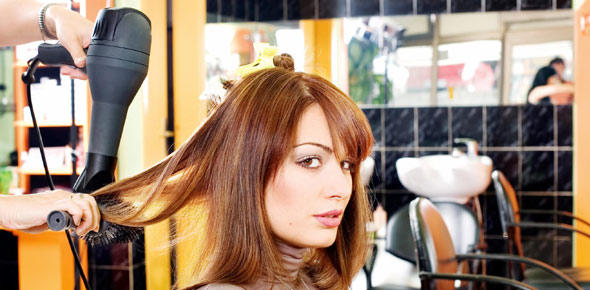Chapter 4 Review Salon Fundamentals

36 Questions | Total Attempts: 132Settings• 1.
The longest wavelengths in the visible spectrum are:
• A.

Red rays

• B.

Violet rays

• C.

Yellow rays

• D.

Ultraviolet rays

• 2.
Which of the following is also known as actinic rays?
• A.

Infrared

• B.

Red

• C.

Ultraviolet

• D.

White

• 3.
The process of forcing an acid or alkali into the skin by applying current to the chemical is called:
• A.

Phoresis

• B.

Anaphoresis

• C.

Cataphoresis

• D.

Iontophoresis

• 4.
Which electrotherapy treatment can cause sebum to be broken down or blackheads to be liquefied, as in deeep-pore cleansing?
• A.

Sinusodial

• B.

Anaphoresis

• C.

Cataphoresis

• D.

Desincrustation

• 5.
If the client is holding the active electrode while the cosmetologist manually stimulates the area being treated, what method of Tesla Current is being used?
• A.

Direct application

• B.

Indirect application

• C.

• D.

General electrification

• 6.
Which of the following is a benefit of using infrared light during a facial?
• A.

Kills bacteria

• B.

Produces Vitamin D

• C.

Increases circulation

• D.

Helps control dandruff

• 7.
Bacteria that causes skin infections can be killed by:
• A.

Infrared light

• B.

Ultraviolet light

• C.

Fluorescent light

• D.

Incandescent light

• 8.
A volt is a unit of electric:
• A.

Strength

• B.

Pressure

• C.

Frequency

• D.

Resistance

• 9.
Which is NOT a way for heat to be transferred from one object to another?
• A.

Phoresis

• B.

• C.

Convection

• D.

Conduction

• 10.
Tesla, a high frequency current, is known as the:
• A.

Red ray

• B.

Violet ray

• C.

Infrared ray

• D.

Ultraviolet ray

• 11.
A constant electric current flowing in one direction is called a(n):
• A.

Direct current

• B.

• C.

Sinusoidal Current

• D.

Alternating current

• 12.
How many milliamperes equal one ampere?
• A.

10

• B.

100

• C.

1000

• D.

1000000

• 13.
Before using ultraviolet light on a client, the skin or scalp should be:
• A.

Wet

• B.

Oily

• C.

Cleansed

• D.

Moisturized

• 14.
Which of the following materials does NOT allow a current to pass through it?
• A.

Metal

• B.

Water

• C.

Carbon

• D.

Alcohol

• 15.
Light, heat, chemical and magnetic changes are all produced by:
• A.

Force

• B.

Conductors

• C.

Electricity

• D.

A short circuit

• 16.
A ampere is a unit of electric:
• A.

Strength

• B.

Pressure

• C.

Frequency

• D.

Resistance

• 17.
High Frequency and alternating current are characteristics of which type of electrotherapy?
• A.

Tesla

• B.

Galvanic

• C.

Sinusoidal

• D.

Direct current

• 18.
Any electrical appliance activated by the flow of electric current such as a blow dryer is known as a(n):
• A.

• B.

Electron

• C.

Insulator

• D.

Conductor

• 19.
Which of the following devices breaks the flow of current when an overload occurs?
• A.

Fuse

• B.

Insulator

• C.

Circuit breaker

• D.

Grounding wire

• 20.
Materials that don't allow electricity to floe through them are called:
• A.

Insulators

• B.

Conductors

• C.

Open circuits

• D.

Closed circuits

• 21.
What temporary effect will a negative electrode pole produce when applying Galvanic Current during an electrotherapy treatment?
• A.

Increase blood flow

• B.

Expansion of nerve tissues

• C.

Contraction of blood vessels

• D.

Production of an acid reaction

• 22.
The application of special currents (or modalities) that have a variety of effects on the skin is called:
• A.

Light therapy

• B.

Electrotherapy

• C.

Chemical therapy

• D.

Mechanical therapy

• 23.
Material that best transports electricity is a(n):
• A.

Watt

• B.

Prism

• C.

Insulator

• D.

Conductor

• 24.
Which type of light can be broken into its individual wavelengths by a prism?
• A.

Infrared

• B.

Ultraviolet

• C.

Combination

• D.

Incandescent

• 25.
Faradic Current is used chiefly to cause:
• A.

Soothing effects

• B.

Chemical effects

• C.

The transfer of heat

• D.

Muscle contractions

Related TopicsBack to top